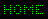206a
<< <> >>

# 206

The programming language Forth### Without exception

When multiplying x and y the answer is y*x, unless 0 or 1 is involved:
```: TIMES ( x y -- y*x | x | y )
OVER 0=  OVER 1 = OR IF DROP EXIT THEN
OVER 1 = OVER 0=  OR IF NIP  EXIT THEN
* ;
```
However, we can simplify this:
```: TIMES ( x y -- y*x ) * ;
```
This illustrates that obvious exceptions not necessarily need special treatment. Of course, it will not always be as simple as TIMES.
The following program removes exceptions ( **) by transforming them to regular actions. Because there are no conditional branches anywhere, the program flow is very easy to follow.
The only concession is the UNTIL at the end of PLAY.
```Forth file: ec206.frt
\ Shift game (Albert Nijhof)
\ The player shifts the blocks to their correct position.

FORTH DEFINITIONS  DECIMAL
: CHAR-ARRAY ( amount -- )      \ Defining word
CREATE 255 UMIN               \ Max. length? ( **)
DUP C, 1+ ALLOT ALIGN
DOES>  ( index -- address )
COUNT ROT UMIN + ;     \ Range?       ( **)

#16 CHAR-ARRAY board       \ 15 chars plus an empty space
0 VALUE SPOT               \ Position of empty space

: FINAL ( -- )